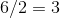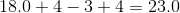# Computer Science : Evaluating Numerical Expressions

## Example Questions

### Example Question #1 : Evaluating Numerical Expressions

int a = 49;

int b = 6;



int c = 49 % 6;

What is the value of c?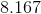Explanation:

The modulus (or modulo) operator returns the remainder of a division statement. It only works with integer operands. Thus, it will only return an integer result. Six is able to divide forty-eight a maximum of eight times.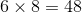And then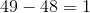Thus,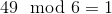### Example Question #1 : Evaluating Numerical Expressions

int a = 5;

a += 3.14;

Using the language C++ or Java, what will the value of a be after the above code snippet?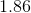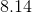Explanation:

When using the plus-equals (+=) operator, the answer is cast to the type of the left-hand argument. Since a was an int, the value returned by the expression is converted to int before it is stored in a. Thus, the decimal point and any information behind the decimal is truncated.

### Example Question #1 : Evaluating Numerical Expressions

Evaluate the mathematical equation?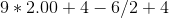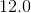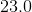Explanation:

You have to remember PEMDAS.Multiplication and Division take precedence first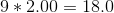(double multiplied by an int equal a double)